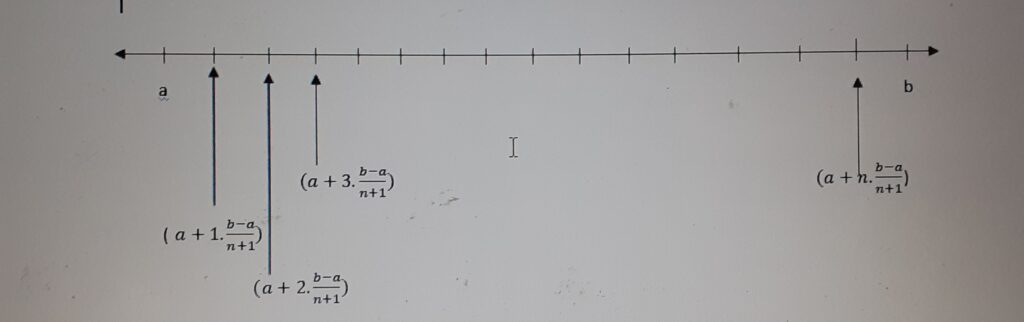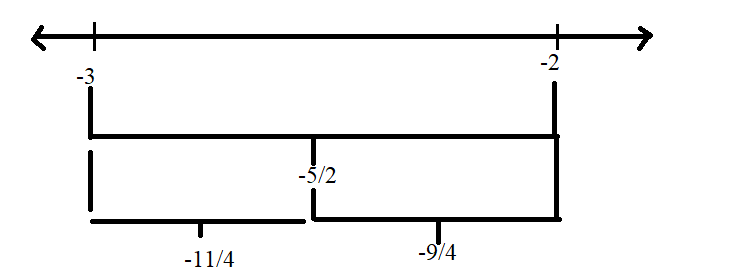September 29, 2023

# Finding rational numbers between two given rational numbers

Rational numbers between two given rational numbers

A rational number is a number which can be written in the form of . We can find  infinitely many rational numbers between any two rational numbers. This property of rational numbers is known as the dense property. Methods to  find  rational numbers between any two rational numbers are given below..

Contents

# Method-1  (Average Technique)

Suppose  we are required to find  rational numbers between two rational numbers

and such that . Since the average of two numbers always  lying between the numbers, so is a rational number lying between   and .  We continously find out the averages of two numbers to find a number in between the first two numbers. We continue this method  until we find out as many rational numbers as we need.

e.g. Find 4 rational numbers between 1 and 2 .

sol. Let and then  is a number between 1 and 2.

Now a number between 1 and  is . We can proceed in this manner to find two more numbers between 1 and 2.  Thus four  rational numbers between 1 and 2 is   and .

## Method 2 (Gap Method)

Finding  rational numbers between any two rational numbers and when . Use the following steps –

Step 1- Find the gap between the  given rational numbers   and (). Gap =

Step 2- Divide the gap by  .

Step 3- Multiply   by and add each product to .

Thus rational numbers between the given rational numbers    and   are

.e.g. Find  6 rational numbers between and .

Sol.Let    and , then    . Gap between and     =    .

To find  6 rational numbers , divide by .

Dividing the gap by 7, we get

Thus the 6 rational numbers between   and are

,    ,    , and   ,

i.e. .

### Method 3 : To find Rational Numbers between Two Given Rational Numbers with the Same Denominator

(i) If the numerators differ by a large value then you can simply write the rational numbers with an increment of one while keeping the denominator part unchanged.

(ii) If the numerators differ by a smaller value than the number of rational numbers to be found simply multiply the numerators and denominators by multiples of 10.

e.g. Suppose we have to find rational numbers between and  .

Obviously     are rational numbers between the given numbers. But we can write  and .  Now the numbers    all are between   and .

Also can be expressed as   and as  . Now we see that are between and .  In this way, we can go on inserting more and more rational numbers between    and  .

So we can find countless  rational numbers between any two given rational numbers.

#### Method 4:  To find Rational Numbers between Two  given Rational Numbers with the Different Denominators

• To find Rational Numbers between Two Rational Numbers with the Different Denominators you need to equate the Denominators firstly.
• You can Equate the Denominators by finding their LCM or by multiplying the denominators of one to another one’s numerator and denominator.

e.g. Find any 10 rational numbers between and  .

Sol.  We  first convert  and   to rational  numbers  with  the same denominator.    and  . Thus we have as rational numbers between   and   .

Some more examples:

e.g. How many rational numbers lie between -1/4 and 1/4?

Sol.  We can write      and .  Now the numbers    all are between and .

Also can be expressed as     and      as  .   Now we see that  are between and .  In this way, we can go on inserting more and more rational numbers between    and  .

So we can find countless  rational numbers between any two given rational numbers.

e.g.(ii)  list three rational numbers between -3 and -2.

sol. Let   and  . Then is a rational number  between -3 and -2.

Now a number between  -3 and    is    . We can proceed in this manner to find one more numbers between -3 and -2. Thus three rational numbers between -3 and -2 are    and .